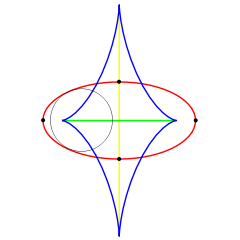# Medial axis

Medial axisAn ellipse (red), its evolute (blue), and its medial axis (green). The symmetry set, a super-set of the medial axis is the green and yellow curves. One bi-tangent circle is shown.

The medial axis of an object is the set of all points having more than one closest point on the object's boundary. Originally referred to as the topological skeleton, it was introduced by Blum  as a tool for biological shape recognition. In mathematics the closure of the medial axis is known as the cut locus.

In 2D, the medial axis of a plane curve S is the locus of the centers of circles that are tangent to curve S in two or more points, where all such circles are contained in S. (It follows that the medial axis itself is contained in S.) The medial axis of a simple polygon is a tree whose leaves are the vertices of the polygon, and whose edges are either straight segments or arcs of parabolas.

The medial axis together with the associated radius function of the maximally inscribed discs is called the medial axis transform (MAT). The medial axis transform is a complete shape descriptor (see also shape analysis), meaning that it can be used to reconstruct the shape of the original domain.

The medial axis is a subset of the symmetry set, which is defined similarly, except that it also includes circles not contained in S. (Hence, the symmetry set of S generally extends to infinity, similar to the Voronoi diagram of a point set.)

The medial axis generalizes to k-dimensional hypersurfaces by replacing 2D circles with k-dimension hyperspheres. The 2D medial axis is useful for character and object recognition, while the 3D medial axis has applications in surface reconstruction for physical models, and for dimensional reduction of complex models.

If S is given by a unit speed parametrisation$\gamma:\mathbf{R}\to\mathbf{R}^2$, and$\underline{T}(t) = {d\gamma\over dt}$ is the unit tangent vector at each point. Then there will be a bitangent circle with center c and radius r if

•$(c-\gamma(s))\cdot\underline{T}(s)=(c-\gamma(t))\cdot\underline{T}(t)=0,$
•$|c-\gamma(s)|=|c-\gamma(t)|=r.\,$

For most curves, the symmetry set will form a one dimensional curve and can contain cusp. The symmetry set has end points corresponding to the vertices of S.(a) A simple 3d object. (b) Its medial axis transform. The colors represent the distance from the medial axis to the object's boundary.

Wikimedia Foundation. 2010.

### См. также в других словарях:

• Medial (disambiguation) — Medial has several meanings: In mathematics, a medial is a set with a binary operation satisfying certain properties, see medial. In anatomy, a medial is an adjective describing structures near the midline of an animal, see anatomical terms of… …   Wikipedia

• medial — [mē′dē əl] adj. [LL medialis < L medius, middle: see MID1] 1. of or in the middle; neither beginning nor ending; median 2. nearer the median plane or axis of a body or part 3. a) of an average or mean b) average; ordinary …   English World dictionary

• Medial geniculate nucleus — Brain: Medial geniculate nucleus Thalamic nuclei: MNG = Midline nuclear group AN = Anterior nuclear group MD = Medial dorsal nucleus VNG = Ventral nuclear group VA = Ventral anterior nucleus VL = Ventr …   Wikipedia

• Medial — The side of the body or body part that is nearer to the middle or center (median) of the body. For example, when referring to the knee, medial would mean the side of the knee that is closest to the other knee The opposite of medial is lateral. *… …   Medical dictionary

• Axis (anatomy) — Bone: Axis (anatomy) Second cervical vertebra, or epistropheus, from above …   Wikipedia

• medial — adjective Etymology: Late Latin medialis, from Latin medius Date: 1570 1. mean, average 2. a. being or occurring in the middle b. extending toward the middle; especially lying or extending toward the median axis of the body 3. situated between… …   New Collegiate Dictionary

• Os cunéiforme médial — Os cunéiforme Emplacement des os cunéïformes Cliquez sur l image pour l agrandir Les os cunéiformes sont présents dans la partie médiale du tarse distal du pied. Comme leur nom l indique, cunéiforme signifie « en forme de coins » (du… …   Wikipédia en Français

• dorsoventral axis — (Subclass Copepoda): An imaginary line through the surface of the body opposite the limbs to the surface bearing the limbs; the terms lateral and medial often are used in place of dorsal and ventral in descriptions of appendages [Ferrari and… …   Crustacea glossary

• reticular nuclei medial column — two groups of reticular nuclei of the brainstem, located symmetrically in the central parts of the two halves of the tegmentum; their neurons are often large, with long axons, and have dendrites and preterminal axons at right angles to the long… …   Medical dictionary

• Topological skeleton — In shape analysis, skeleton (or topological skeleton) of a shape is a thin version of that shape that is equidistant to its boundaries. The skeleton usually emphasizes geometrical and topological properties of the shape, such as its connectivity …   Wikipedia

### Поделиться ссылкой на выделенное

##### Прямая ссылка:
Нажмите правой клавишей мыши и выберите «Копировать ссылку»